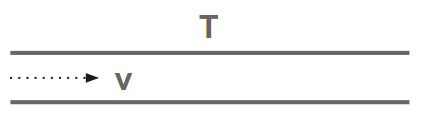Section 13

# The Experiment Of FizeauWe can move clocks and measuring-rods only with velocities that are small compared with the velocity of light. Hence, we shall hardly be able to compare the results of the previous section directly with the reality.

But, on the other hand, these results must strike you as being very singular. This is why I draw another conclusion from the theory.

In Section 6, we derived the theorem of the addition of velocities in one direction in the form which also results from the hypotheses of classical mechanics. This theorem can also be deduced readily from the Galilei transformation (Section 11).

In place of the man walking inside the carriage, we introduce a point moving relatively to the moving K’ according to the equation:

``````x' = wt'
``````

Through the 1st and 4th equations of the Galilei transformation, we can express `x'` and `t'` in terms of `x` and `t`. We then obtain:

``````x = (v + w)t
``````

This equation expresses the law of motion of the point with reference to non-moving `K`. Its velocity is `W`. We then obtain, as in Section 6,

``````W = v + w
``````

We apply the theory of relativity to get=

``````x' = wt'
``````

We must then express `x'` and `t'` in terms of `x` and `t`, making use of the 1st and 4th equations of the Lorentz transformation. Instead of the equation (A) we then obtain the equation:

``````W = (v + w) / 1+((v w)/c2)
``````

which corresponds to the theorem of addition for velocities in one direction according to the theory of relativity. The question now arises as to which of these two theorems is the better in accord with experience.

The brilliant physicist Fizeau did an experiment that made light travel in a motionless liquid with velocity `w`.How quickly does light travel in tube `T` when the liquid is flowing through the tube with a velocity `v`?

Relativity says that light always has the same velocity `w` with respect to the liquid, whether the liquid is moving or not.

The known information are=

• the velocity of light relative to the liquid
• the velocity of the liquid relative to the tube

What is the velocity of light relative to the tube?

The problem of Section 6 again confronts us.

• The tube is the non-moving `K` or the embankment
• The liquid is the moving `K'` or the train
• The light is the man walking in the train, or the moving point in the present.

The velocity of the light `W` relative to the tube this is given by either=

• equation A according to the Galilei transformation or
• equation B according to the Lorentz transformation derived from the theory of relativity

The experiment* shows that the speed is in favour of equation B.

*Einstein note: Fizeau found W = w + v (1 − 1 /n2), where n = c/w is the index of refraction of the liquid. On the other hand, owing to the smallness of vw as compared with 1, we can replace (B) in the first place by W = (w + v)(1 - (vw)/c2 which agrees with Fizeau’s result.

According to recent and most excellent measurements by Zeeman, the influence of the velocity of the liquid flow `v` on the speed of light is represented by formula B to within 1%.

Nevertheless, H. A. Lorentz gave a theory for this phenomenon long before my theory of relativity. His theory was of a purely electrodynamical nature based on a hypotheses that matter has an electromagnetic structure. *

*Superphysics note: We agree with Lorentz that Lorentz theory only applies to the electromagnetic layer and not to the material layer which works according to Newton’s laws. General Relativity therefore does not apply to spacetime. Rather, general relativity merely explains electromagnetism under the influence of spacetime*Superphysics note: Here, Einstein exposes that his theory is really from Lorentz, with a mere ’transformation’ tweak of unifying space and time through the wave-particle duality of light. Einstein’s transformation is dangerous because it gives electromagnetic properties to spacetime, effectively limiting it. In Superphysics, the unity of spacetime is not sourced from light or electromagnetism, but from the aether, which is above spacetime and two layers above electromagnetism (see below). This is similar to Lorentz sourcing the cause of his transformations to the aether. Lorentz’ mistake was to think that the aether was in the spacetime layer, and not above it.#### Latest Articles

Supersociology
##### The Age of the Universe
Material Superphysics
Supereconomics
##### The Elastic Theory of Gravity
Material Superphysics

#### The Simplified Series